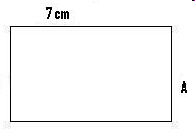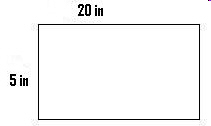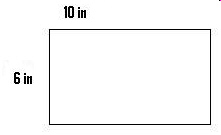Name:    3.MD.7.D.-Test1C Recognize area as additive.

Multiple Choice
Identify the choice that best completes the statement or answers the question.

1.

The formula to find the area of a rectangle is a = _____________.
 a. l ÷ w c. l x w b. l + w d. l - w

2.

Area is the amount of surface a shape _________________.
 a. covers c. moves b. weighs d. none of these

3.

Area is normally measured in square ____________.
 a. lengths c. weights b. digits d. units

4.

Area is normally measured in square ____________.
 a. lengths c. weights b. digits d. units

Completion
Complete each statement.

5.

If the area of the rectangle is 28 sq cm, what is the length of side A? (Use only a number.)6.

If the area of the rectangle is 20 sq in, what is the length of side A?  (Use only a number.)7.

What is the area of the rectangle? (Include the correct area abbreviated identifer - sq m, sq cm, sq in, etc.) NO PERIODS.8.

What is the area of the rectangle? (Include the correct area abbreviated identifer - sq m, sq cm, sq in, etc.) NO PERIODS.9.

What is the area of the rectangle? (Include the correct area abbreviated identifer - sq m, sq cm, sq in, etc.) NO PERIODS.10.

What is the area of the rectangle? (Include the correct area abbreviated identifer - sq m, sq cm, sq in, etc.) NO PERIODS.